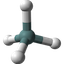Molecular Exact Mass Calculator

QHow to calulate the exact mass of molecule from a formula?

To help you calculating the exact mass for a given molecular formula, FYIcenter.com has designed this online tool. All you need to do is to enter a molecular formula, and click the "Go" button below.

The exact mass of molecule will be presented in the result area below:

Molecular Formula:

✍: FYIcenter.com

A

Output by FYIcenter.com on Your Molecular Formula:

Specified Molecular Formula: Valid

```Molecular Formula: C8H10N4O2
Exact Mass:
194.08 u (atom mass unit)
```

What is exact mass of a molecule?

Exact mass of a molecule is the sum of the atomic mass of the most common isotope of atoms in a given molecule. The most accurate atomic isotope mass available on the Internet is used in this calculator.

There is no periodic table that includes the most common isotopic mass. But many of them include average isotopic mass based on abundances as shown below: